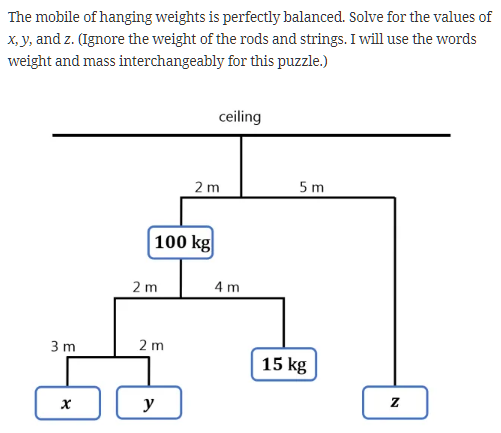# 一点儿有意义的事: SICP in Elisp

SICP常读常新, 如绘画, 层层递进, 构图, 起形, 框架, 明暗, 光线… 反复阅读, 逐次夯建, 领会书中妙处.

9赞

SICP 1.3.1 黎曼积分公式

``````(define (integral f a b dx)
(define (add-dx x) (+ x dx))
(* (sum f (+ a (/ dx 2.0)) add-dx b)
dx))
``````

``````#+name: case-1.3.1-integral.scm
#+BEGIN_SRC scheme :session sicp

(define (f b)
(lambda (x) (/ 1 (sqrt
(- (sin x)
(sin b))))))

(define pi 3.141592653589793)

(define (integral2 f a b dx)
(define (add-dx x) (+ x dx))
(* (sum (f b)
(+ a (/ dx 2.0))
(lambda (x) (+ x dx))
b)
dx))

(* (integral2 f 0 (/ pi 6) 0.00001)
(sqrt (/ 40
(* 3 9.8))))
#+END_SRC

#+RESULTS: case-1.3.1-integral.scm
: 0.0-1.777598336021436i
``````

1.777 vs 1.78

Exercise 1.5[x]

Ben Bitdiddle has invented a test to determine whether the interpreter he is faced with is using applicative-order evaluation or normal-order evaluation. He defines the following two procedures:

``````#+BEGIN_SRC elisp
(defun p (p)) ;; def foo(): return foo()

(defun test(x y)
(if (= x 0)
0
y))
#+END_SRC
``````

Exercise 1.6.[X]

Alyssa P. Hacker doesn’t see why if needs to be provided as a special form. ``Why can’t I just define it as an ordinary procedure in terms of cond?’’ she asks. Alyssa’s friend Eva Lu Ator claims this can indeed be done, and she defines a new version of if:

``````#+name: case-1.1.7-ternamy.el
#+begin_src emacs-lisp :session sicp :lexical t :results none
;; ternamy conditions
(defun new-if (predicate then-clause else-clause)
(cond (predicate then-clause)
(t else-clause)))
#+end_src
``````

Delighted, Alyssa uses new-if to rewrite the square-root program: What happens when Alyssa attempts to use this to compute square roots? Explain.

``````#+begin_src emacs-lisp :session sicp :lexical t
(defun sqrt-iter(guess x)
(new-if (good-enough-p guess x)
guess
(sqrt-iter (improve guess x)
x)))
(sqrt-iter 1 10)

#+end_src
``````

Exercise 1.34[x]

Suppose we define the procedure．

#+BEGIN_SRC scheme (define (f g) (g 2)) (f square) #+END_SRC

``````#+RESULTS:

: (f (lambda (z) (* z (+ z 1))))
6
``````

What happens if we (~perversely~) ask the interpreter to evaluate the combination (f f)? Explain.

SICP中的习题与正文遥相呼应, 习题既是前文的总结又是后文的引子.

02. Abstraction with data

2.3.1 思路清晰的微分求导公式

``````#+name:  case-2.3.1-symbolic-differentiationl.el
#+begin_src emacs-lisp :session sicp :lexical t
(defun deriv(exp var) ;;v
(cond ((numberp exp) 0) ;
((variable-p exp)
(if (same-variable-p exp var) 1 0)) ;;else 0
((sum-p exp)
(make-sum (deriv (addend exp) var) ;;
(deriv (augend exp) var)))
((product-p exp)
(make-sum
(make-product (multiplier exp)
(deriv (multiplicand exp) var))
(make-product (deriv (multiplier exp) var)
(multiplicand exp))))
(t
(error "unknown expression type -- DERIV" exp))))

;; variable
(defun variable-p(x) (symbolp x))
(defun same-variable-p(v1 v2)
(and (variable-p v1) (variable-p v2) (equal v1 v2)))
;; constructor for sum and product
(defun make-sum(a1 a2) (list '+ a1 a2))
(defun make-product(m1 m2) (list '* m1 m2))
;; predicate and selectors for sum
(defun sum-p(x)
(and (consp x) (equal (car x) '+)))
;; predicate and seletors for product
(defun product-p(x)
(and (consp x) (equal (car x) '*)))
#+end_src

#+RESULTS: summary
: multiplicand

#+begin_src emacs-lisp :tangle yes  :results output
(print (deriv '(+ x 3) 'x))
(print (deriv '(* x y) 'x))
(print (deriv '(* (* x y) (+ x 3)) 'x))
#+end_src
#+RESULTS:

:
: (+ 1 0)
:
: (+ (* x 0) (* 1 y))
:
: (+ (* (* x y) (+ 1 0)) (* (+ (* x 0)``````

https://docs.racket-lang.org/racket-cheat/index.html#(section._.Essentials)

https://docs.racket-lang.org/guide/index.html

``````(org-babel-do-load-languages 'org-babel-load-languages '(

(scheme . t)

))
``````

`````` sudo apt install racket
``````

``````(add-hook 'scheme-mode-hook 'geiser-mode)
(setq geiser-default-implementation 'racket)
``````

OK了.

``````#+begin_src scheme :session sicp
(* 3 3)
#+end_src

#+RESULTS:
: 9``````
1赞
1赞

Exercise 2.29. A binary mobile consists of two branches, a left branch and a right branch. Each branch is a rod of a certain length, from which hangs either a weight or another binary mobile. We can represent a binary mobile using compound data by constructing it from two branches (for example, using list):(家里的风铃

1赞